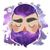# App Development

Discussion board where members can learn more about Qlik Sense App Development and Usage.

Announcements
April 22, 2PM EST: Learn about GeoOperations in Qlik Sense SaaS READ MORE
cancel
Showing results for
Search instead for
Did you mean:Contributor

## Color a Cell in a Table based on value from Formula

Hello, I'm struggling with the below. The formula works perfect as a Master Measure, what I want to do is highlight the cell in RED if the answer is below 120 and GREEN if equal to 120 or greater.

" IF((((((Sum({<TCODE={'IN'}>} AMOUNT))-(Sum({<TCODE={'CN'}>} AMOUNT)))-((Sum({<TCODE={'IN'}>} PROFIT))-(Sum({<TCODE={'CN'}>} PROFIT))))/(SUM(ONHAND*LTSTCOST)))*(((Sum({<TCODE={'IN'}>} PROFIT))-(Sum({<TCODE={'CN'}>} PROFIT)))/((Sum({<TCODE={'IN'}>} AMOUNT))-(Sum({<TCODE={'CN'}>} AMOUNT))))*100)>'120', 'RED',) "

Labels (4)

• ### table

0 Replies